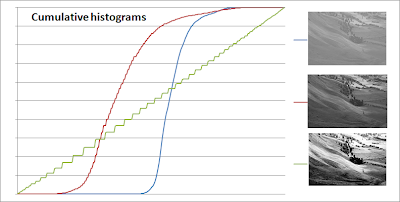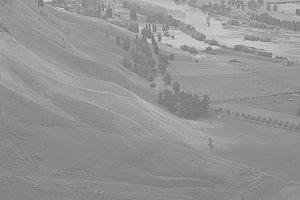## Monday, January 24, 2011

### Algorithm to determine image contrast

How to determine image contrast ? More specifically - How to determine that image contrast is low and it needs automatic contrast adjustment through histogram equalization method ?

Algorithm is this:
1. Calculate cumulative histogram of image.
2. Make linear regression of cumulative histogram in the form freq(x) = A*x + B.
3. Calculate RMSE of real_cumulative_frequency(x)-freq(x).
4. If that RMSE is close to zero - image is already equalized and should be in good contrast. (That means for equalized images cumulative histograms must be linear)

Because picture is worth a thousand words - this is how cumulative histograms looks for image with different levels of contrast:So as you see - image with most aggressive contrast has cumulative histogram which is almost a perfect line.

Now fun part - C code which opens image in PGM format with ASCII encoding and detects if image needs contrast adjustment or not :
```#include <stdio.h>
#include <stdlib.h>
#include <math.h>
#include <string.h>

#define MAX_COLS 1000
#define MAX_ROWS 1000

typedef struct {
int Width;
int Height;
int max_value;
int data[MAX_ROWS][MAX_COLS];
} PGMdata;

void readPgmFile(char* fileName, PGMdata * pgmOut) {
FILE * pFile;
char line;
char* res;
int lineNum = 0;

if ((pFile = fopen(fileName , "r")) == NULL)
printf("Error opening file %s \n", fileName);
else {
while ((res = fgets(line, 100, pFile)) != NULL) {
lineNum++;
// max value of pixel
if (lineNum==4)
sscanf(line,"%i",&pgmOut->max_value);
// width and height of image
if (lineNum==3) {
sscanf(line,"%i %i",&pgmOut->Width, &pgmOut->Height);
}
if (lineNum > 4) {
int ix = lineNum - 5;
int row = ix/pgmOut->Width;
int col = ix - row*pgmOut->Width;
sscanf(line,"%i", &pgmOut->data[row][col]);
}
}
fclose(pFile);
}
}

void CumulativeHistogram(PGMdata* image, double* hist) {
int i,j;
double dp = 1.0/((double) image->Width*image->Height);

// initializing histogram bins to zero
for (i=0; i<256; i++) {
hist[i] = 0.0;
}

// calculating histogram
for (i=0; i<image->Width; i++) {
for (j=0; j<image->Height; j++) {
hist[image->data[j][i]] += dp;
}
}

// making histogram cumulative
for (i=0; i<255; i++) {
hist[i+1] += hist[i];
}
}

void CreateXmatrix(double mat) {
int i;
for (i=0; i<256; i++) {
mat[i] = 1;
mat[i] = i;
}
}

void TransposeMatrix(double mat, double tmat) {
int i,j;
for (i=0; i<2; i++) {
for (j=0; j<256; j++) {
tmat[i][j] = mat[j][i];
}
}
}

double MultiplyMatrixes(double A, double B, int row, int col) {
int i;
double sum = 0.0;

for (i=0; i<256; i++) {
sum += A[row][i]*B[i][col];
}

return sum;
}

double MultiplyMatrixAndVector(double A, double Y, int row) {
int i;
double sum = 0.0;

for (i=0; i<256; i++) {
sum += A[row][i]*Y[i];
}

return sum;
}

double HistogramPredicted(double c0, double c1, double level) {
return c0 + c1*level;
}

double RootMeanSquare(double* hist, double c0, double c1) {
double rms = 0.0;
int i;

for (i=0; i<256; i++) {
rms += pow(hist[i]-HistogramPredicted(c0,c1,i),2.0);
}
rms /= 256.0;

return sqrt(rms);
}

void LinearLeastSquares(double* hist, double* c0, double* c1) {
double X;
double tX;
double a1,a2,a3,a4;
double b1, b2;

// create matrix X composed of x'es
CreateXmatrix(X);

// transpose X matrix
TransposeMatrix(X,tX);

// calculate tX*X matrix which is
// [a1 a2]
// [a3 a4]

a1 = MultiplyMatrixes(tX,X,0,0);
a2 = MultiplyMatrixes(tX,X,0,1);
a3 = MultiplyMatrixes(tX,X,1,0);
a4 = MultiplyMatrixes(tX,X,1,1);

// calculate tX*Y  (Y=HISTOGRAM) which is
// [b1]
// [b2]

b1 = MultiplyMatrixAndVector(tX,hist,0);
b2 = MultiplyMatrixAndVector(tX,hist,1);

// solve matrix equation by using elimination of variables method
// [a1 a2]   [c0]   [b1]
//         *      =
// [a3 a4]   [c1]   [b2]

*c1 = (a1*b2-a3*b1)/(a1*a4-a2*a3);
*c0 = (b1-a2*(*c1))/a1;

}

int main () {
double hist;
static PGMdata image = {0};
double c0=0.0, c1=0.0;
double rms;
char pgm_file = {0};

while (1) {
// get PGM file name
printf("PGM filename: ");
scanf("%s", pgm_file);

// read grayscale image from PGM format
memset(&image, 0, sizeof(image));
if (image.Width == 0)
continue;

// create cumulative histogram
CumulativeHistogram(&image, hist);

// least mean squares method, to find c0,c1 coefficents in equation:
// c0 + c1*gray_level = Frequency
LinearLeastSquares(hist,&c0,&c1);

// calculate RMS of Frequency[bin]-predicted_Frequency(bin)
rms = RootMeanSquare(hist,c0,c1);

// Low RMS shows that histogram frequencies are distributed in linear fashion
// between bins. This means that histogram equalization was performed on image
// and/or that global image contrast is good.
if (rms <= 0.01)
printf("\n==> %s contrast is OK (rms=%f)\n\n", pgm_file ,rms);
else
printf("\n==> %s contrast is BAD (rms=%f)\n\n", pgm_file ,rms);
}

return 0;
}
```
If you want to test this algorithm on ASCII PGM image - you better covert image to PGM format with GIMP 2.6.11, because it was only tested in this way.
So by running this C code on below image (after converting it to PGM)we get algorithm output
"image contrast is BAD ... " :-)

1.2.